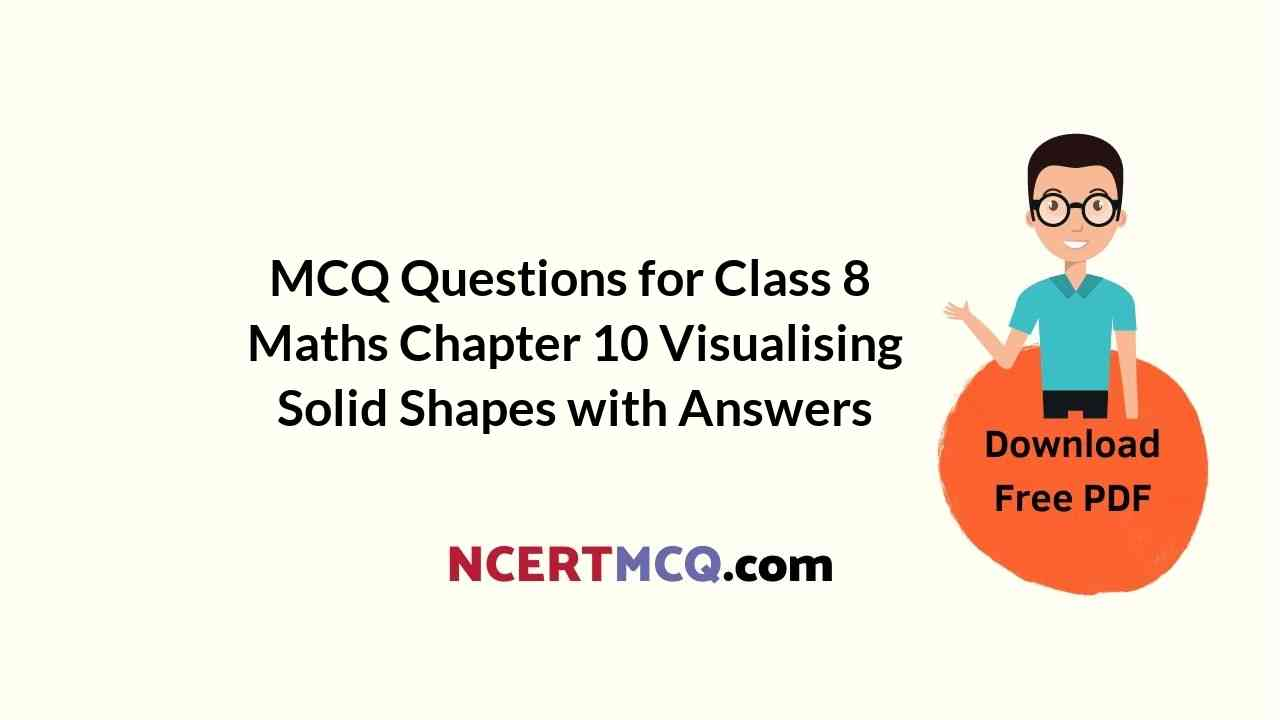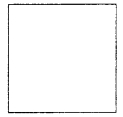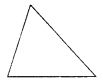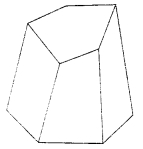Check the below Online Education NCERT MCQ Questions for Class 8 Maths Chapter 10 Visualising Solid Shapes with Answers Pdf free download. MCQ Questions for Class 8 Maths with Answers were prepared based on the latest exam pattern. We have provided Visualising Solid Shapes Class 8 Maths MCQs Questions with Answers to help students understand the concept very well. https://ncertmcq.com/mcq-questions-for-class-8-maths-with-answers/

Students can also refer to NCERT Solutions for Class 8 Maths Chapter 10 Visualising Solid Shapes for better exam preparation and score more marks.

## Online Education for Visualising Solid Shapes Class 8 MCQs Questions with Answers

Visualising Solid Shapes Class 8 MCQ Question 1.
The name of the shape is(a) cylinder
(b) square
(c) circle
(d) triangle.

MCQ On Visualising Solid Shapes Class 8 Question 2.
The name of the shape is(a) sphere
(b) cylinder
(c) cone
(d) triangle.

Class 8 Maths Chapter 10 MCQ Question 3.
The name of the shape is(a) cone
(b) sphere
(c) cuboid
(d) cylinder.

Ncert Class 8 Maths Chapter 10 MCQ Question 4.
The name of the shape(a) triangle
(b) cone
(c) cylinder
(d) sphere

Class 8 Maths Ch 10 MCQ Question 5.
The name of the shape is(a) cone
(b) circle
(c) cylinder
(d) cube.

Class 8 Visualising Solid Shapes MCQ Question 6.
The name of the shape is(a) cuboid
(b) cube
(c) square
(d) cylinder.

MCQ Questions For Class 8 Maths Visualising Solid Shapes Question 7.
The name of the shape is(a) cube
(b) cuboid
(c) sphere
(d) square.

Visualising Solid Shapes MCQ Question 8.
The name of the shape is(a) circle
(b) sphere
(c) cylinder
(d) cone.

Class 8 Maths Chapter 10 Extra Questions Question 9.
A cuboid has how many faces ?
(a) 2
(b) 4
(c) 6
(d) 3.

Visualising Solid Shapes Class 8 Extra Questions Question 10.
How many faces does a cube have ?
(a) 6
(b) 4
(c) 3
(d) 2.

Class 8 Maths Chapter 10 MCQ Questions Question 11.
How many vertices does a cuboid have ?
(a) 4
(b) 6
(c) 8
(d) 3.

Visualising Solid Shapes MCQ Class 8 Question 12.
How many vertices does a cube have ?
(a) 8
(b) 6
(c) 4
(d) 2.

The Side View Of A Cone Appears As Question 13.
How many edges does a cuboid have ?
(a) 12
(b) 8
(c) 6
(d) 4.

Class 8th Maths Chapter 10 MCQ Question 14.
How many edges does a cube have ?
(a) 12
(b) 6
(c) 4
(d) 8.

Class 8 Math Chapter 10 MCQ Question 15.
How many faces does a triangular pyramid have ?
(a) 1
(b) 2
(c) 3
(d) 4.

MCQ Questions For Class 8 Maths Chapter 10 Question 16.
How many vertices does a triangular pyramid have ?
(a) 1
(b) 2
(c) 3
(d) 4.

MCQ Questions On Visualising Solid Shapes Class 8 Question 17.
How many edges does a triangular pyramid have ?
(a) 2
(b) 4
(c) 6
(d) 8.

Question 18.
How many faces does a triangular prism have ?
(a) 5
(b) 4
(c) 3
(d) 6.

Question 19.
How many vertices does a triangular prism have ?
(a) 6
(b) 5
(c) 4
(d) 3.

Question 20.
How many edges does a triangular prism have ?
(a) 11
(b) 9
(c) 6
(d) 3.

Question 21.
How many faces does a pyramid with square base have ?
(a) 5
(b) 3
(c) 2
(d) 6.

Question 22.
How many vertices does a pyramid with square base have ?
(a) 5
(b)4
(c) 3
(d) 6.

Question 23.
How many edges does a pyramid with square base have ?
(a) 8
(b) 6
(c) 4
(d) 5.

See the following solid and answer the following questions:Question 24.
The number of faces is
(a) 6
(b) 7
(c) 8
(d) 9.

Question 25.
The number of vertices is
(a) 5
(b) 7
(c) 8
(d) 10

Question 26.
The number of edges is
(a) 5
(b) 10
(c) 20
(d) 15.

Question 27.
For a polyhedron, if ‘F’ stands for number of faces, V stands for number of vertices and E stands for number of edges, then which of the following relationships is named as Euler’s formula ?
(a) F + V = E + 2
(b) F + E = V + 2
(c) V + E = F + 2
(d) F+ V = E – 2.

Answer: (a) F + V = E + 2

Question 28.
A cube has ______ faces.
(a) 4
(b) 5
(c) 6
(d) 8

Explanation:
A cube is a three dimensional shape having six faces.

Question 29.
A sphere has how many faces ?
(a) One
(b) Two
(c) Four
(d) None

Explanation:
A sphere is a round-shaped object having no faces and edges.

Question 30.
The top-view of a cone looks like:
(a) A Circle
(b) A Square
(c) A Rectangle
(d) A Triangle

Explanation:
The base of a right-cone is always circular. When we see it from the top, it looks like a circle with its vertex as the center of the circle.

Question 31.
The top-view of a cube looks like a:
(a) Circle
(b) Square
(c) Rectangle
(d) Triangle

Explanation:
Since all the faces of a cube are of equal length.

Question 32.
The base of a prism is:
(a) Circle
(b) Triangle
(c) Square
(d) Any shape

Explanation:
A pyramid is a polyhedron whose base is a polygon (of any number of sides) and whose lateral faces are triangles with a common vertex.

Question 33.
If a polyhedron has 6 vertices and 12 edges. What is the number of faces it has ?
(a) 6
(b) 8
(c) 12
(d) 18

Explanation:
By Euler’s formula we know that:
For any polyhedron,
F + V – E = 2
F = 2 – V + E
F = 2 – 6 + 12 = 8

Question 34.
Number of vertices a cone has:
(a) One
(b) Two
(c) Three
(d) Four

Question 35.
An example of cone is:
(a) Tube
(b) A tent
(c) A ball
(d) A box

Question 36.
The top-view of a cuboid looks like a:
(a) Circle
(b) Square
(c) Rectangle
(d) Triangle

Question 37.
The side-view of a cone appears as:
(a) Circle
(b) Square
(c) Rectangle
(d) Triangle